Courses

# Test: Electromagnetic Induction (Competition Level 1)

## 30 Questions MCQ Test Exclusive Video Lectures of Class 12 Physics by Experts | Test: Electromagnetic Induction (Competition Level 1)

Description
This mock test of Test: Electromagnetic Induction (Competition Level 1) for Class 12 helps you for every Class 12 entrance exam. This contains 30 Multiple Choice Questions for Class 12 Test: Electromagnetic Induction (Competition Level 1) (mcq) to study with solutions a complete question bank. The solved questions answers in this Test: Electromagnetic Induction (Competition Level 1) quiz give you a good mix of easy questions and tough questions. Class 12 students definitely take this Test: Electromagnetic Induction (Competition Level 1) exercise for a better result in the exam. You can find other Test: Electromagnetic Induction (Competition Level 1) extra questions, long questions & short questions for Class 12 on EduRev as well by searching above.
QUESTION: 1

### A flux of 1m Wb passes through a strip having an area A = 0.02 m2. The plane of the strip is at an angle of 60º to the direction of a uniform field B. The value of B is

Solution: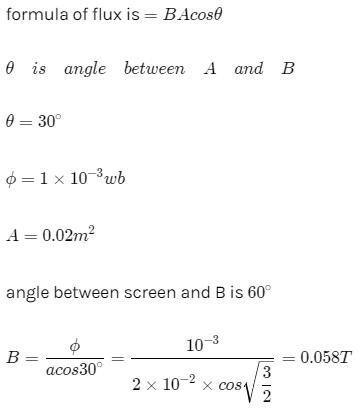QUESTION: 2

Solution:
QUESTION: 3

### A conducting loop of radius R is present in a uniform magnetic field B perpendicular the plane of the ring. If radius R varies as a function of time `t', as R = R0 + t. The e.m.f induced in the loop is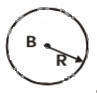Solution:
QUESTION: 4

The instantaneous flux associated with a closed circuit of 10Ω resistance is indicated by the following reaction f = 6t2 _ 5t + 1, then the value in amperes of the induced current at t = 0.25 sec will be

Solution:
QUESTION: 5

When a magnet is being moved towards a coil, the induced emf does not depend upon

Solution:
QUESTION: 6

An electron is moving in a circular orbit of radius R with an angular acceleration a. At the centre of the orbit is kept a conducting loop of radius r, (r < < R). The e.m.f induced in the smaller loop due to the motion of the electron is

Solution:
QUESTION: 7

A field of 5 x 104/p ampere-turns/metre acts at right angles to a coil of 50 turns of area 10-2 m2. The coil is removed from the field in 0.1 second. Then the induced emf in the coil is

Solution: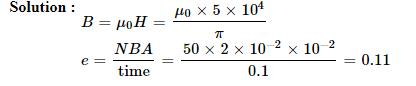QUESTION: 8

square wire loop of 10.0 cm side lies at right angles to a uniform magnetic field of 20T. A 10 V light bulb is in a series with the loop as shown in the fig. The magnetic field is decreasing steadily to zero over a time interval Dt. The bulb will shine with full brightness if Dt is equal to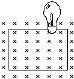Solution:
QUESTION: 9

The rate of change of magnetic flux denisty through a circular coil of area 10-2 m2 and number of turns 100 is 103 Wb/m2/s. The value of induced e.m.f. will be

Solution: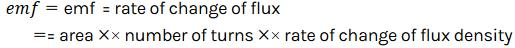QUESTION: 10

A long solenoid contains 1000 turns/cm and an alternating current of peak value 1A is flowing in it. A search coil of area of cross-section 1 × 10-4 m2 and having 50 turns is placed inside the solenoid with its plane perpendicular to the axis of the solenoid. A peak voltage of 2p2 × 10-2V is produced in the search coil. The frequency of current in the solenoid will be

Solution:
QUESTION: 11

In an iron cored coil the iron core is removed so that the coil becomes an air cored coil. The inductance of the coil will

Solution:
QUESTION: 12

The magnetic flux through a stationary loop with resistance R varies during interval of time T as f = at (T _ t). The heat generated during this time neglecting the inductance of loop will be

Solution:
QUESTION: 13

The dimensions of permeability of free space can be given by

Solution:
QUESTION: 14

A closed planar wire loop of area A and arbitrary shape is placed in a uniform magnetic field of magnitude B, with its plane perpendicular to magnetic field. The resistance of the wire loop is R. The loop is now turned upside down by 180° so that its plane again becomes perpendicular to the magnetic field. The total charge that must have flowen through the wire ring in the process is

Solution:
QUESTION: 15

A square coil ABCD is placed in x-y plane with its centre at origin. A long straight wire, passing through origin, carries a current in negative z-direction. Current in this wire increases with time.The induced current in the coil is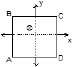Solution:
QUESTION: 16

Two identical coaxial circular loops carry a current i each circulating in the same direction. If the loops approach each other

Solution:
QUESTION: 17

In the arrangement shown in given figure current from A to B is increasing in magnitude. Induced current in the loop will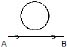Solution:
QUESTION: 18

The north pole of a magnet is brought near a coil. The induced current in the coil as seen by an observer on the side of magnet will be

Solution:
QUESTION: 19

A metal sheet is placed in a variable magnetic field which is increasing from zero to maximum. Induced current flows in the directions as shown in figure. The direction of magnetic field will be -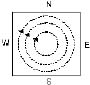Solution:
QUESTION: 20

A small conducting rod of length l, moves with a uniform velocity v in a uniform magnetic field B as shown in fig-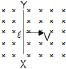Solution:
QUESTION: 21

The magnetic field in a region is given by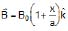. A square loop of edge-length d is placed with its edge along x & y axis. The loop is moved with constant velocity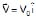. The emf induced in the loop is

Solution:
QUESTION: 22

Consider the situation shown in fig. The resistanceless wire AB is slid on the fixed rails with a constant velocity. If the wire AB is replaced by a resistanceless semicircular wire, the magnitude of the induced current will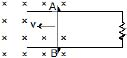Solution:
QUESTION: 23

A conducting square loop of side I and resistance R moves in its plane with a uniform velocity v perpendicular to one of its sides. A uniform and constant magnetic field B exists along the perpendicular to the plane of the loop in fig. The current induced in the loop is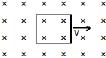Solution:

Voltage is induced due to change in the magnetic flux through a closed loop.
Here
Magnetic Flux = ϕ=BA=const.
Hence
Induced EMF = dtdϕ​=0
Induced current =i=E/R=0

QUESTION: 24

Two identical conductors P and Q are placed on two frictionless fixed conducting rails R and S in a uniform magnetic field directed into the plane. If P is moved in the direction shown in figure with a constant speed, then rod Q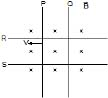Solution:
QUESTION: 25

Two infinitely long conducting parallel rails are connected through a capacitor C as shown in the figure. A conductor of length l is moved with constant speed v0. Which of the following graph truly depicts the variation of current through the conductor with time ?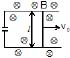Solution:
QUESTION: 26

A thin wire of length 2m is perpendicular to the xy plane. It is moved with velocity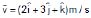through a region of magnetic induction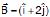Wb/m2. Then potential difference induced between the ends of the wire

Solution:
QUESTION: 27

A long metal bar of 30 cm length is aligned along a north south line and moves eastward at a speed of 10 ms-1. A uniform magnetic field of 4.0 T points vertically downwards. If the south end of the bar has a potential of 0V, the induced potential at the north end of the bar is

Solution:
QUESTION: 28

A conducting rod moves with constant velocity v perpendicular to the long, straight wire carrying a current I as shown compute that the emf generated between the ends of the rod.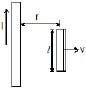Solution:
QUESTION: 29

A square loop of side a and resistance R is moved in the region of uniform magnetic field B (loop remaining completely insidefield), with a velocity v through a distance x. The work done is

Solution: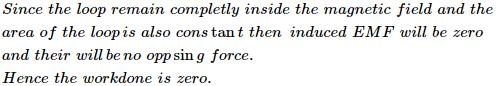QUESTION: 30

A rod closing the circuit shown in figure moves along a U shaped wire at a constant speed v under the action of the force F. The circuit is in a uniform magnetic field perpendicular to the plane. Calculate F if the rate of heat generation in the circuit is Q.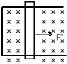Solution: